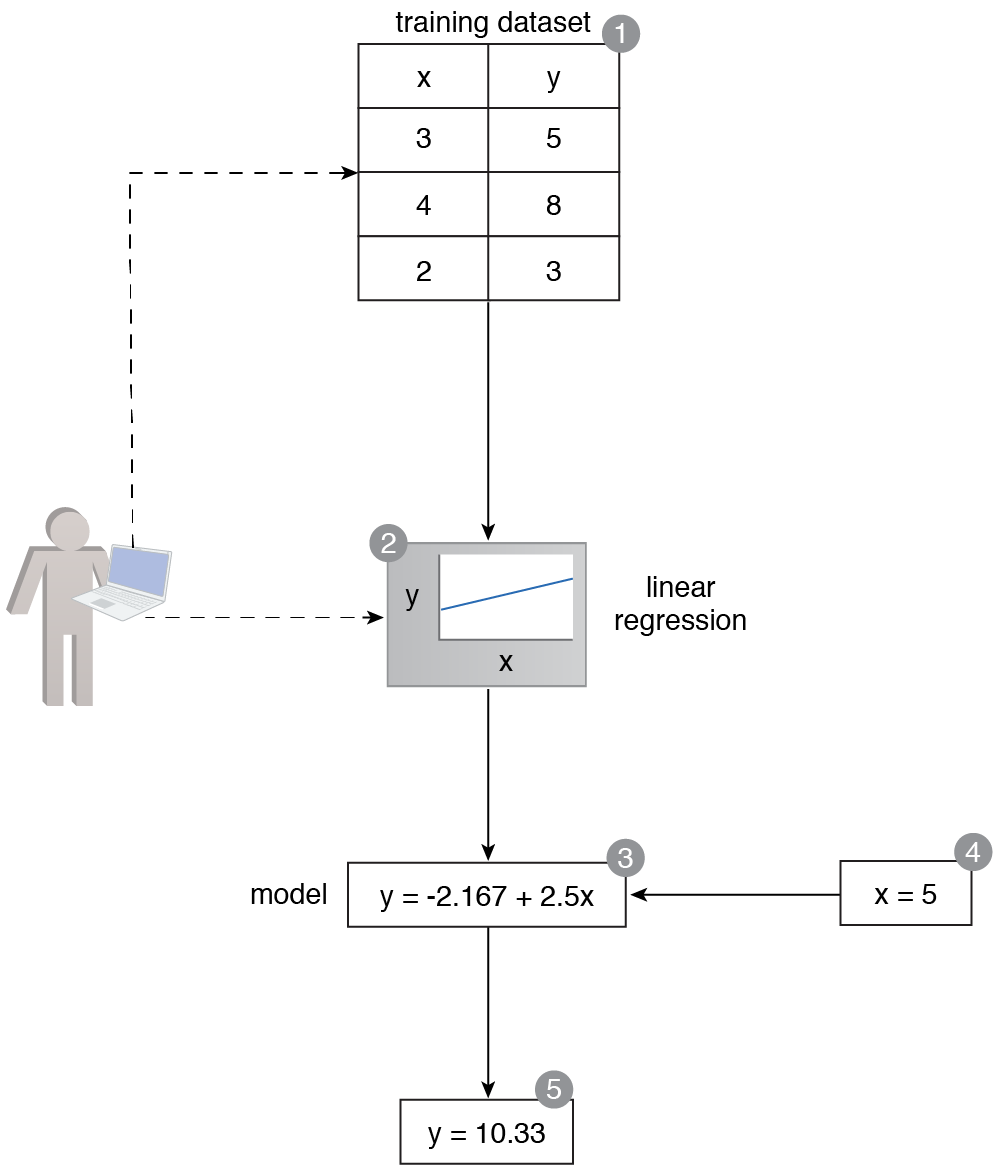# Numerical Prediction

Machine Learning Patterns, Mechanisms > Supervised Learning Patterns > Numerical Prediction

## Numerical Prediction (Khattak)

How can the value of a numerical variable be predicted based on a set of known values of other variables?

### Problem

Certain machine learning problems require predicting a numerical value based on some known variables, making it impossible to apply machine learning techniques where the target can only be a categorical variable.

### Solution

The relationship between the known variables and the target variable is captured using a regression algorithm, which is then used to predict the unknown value of the target variable based on known values of the other variables.

### Application

Regression algorithms, such as linear regression, are applied to the dataset by first developing a model based on the known set of values of both the target and other variables, and then making predictions for the unknown value of the target variable based on a known set of values of other variables.

### Mechanisms

Query Engine, Analytics Engine, Processing Engine, Resource Manager, Storage Device, Visualization EngineA training dataset is comprised of two variables (x and y) that are numerical in nature (1). An algorithm is applied to train a model to predict numerical values (2). The trained model exists in the form of a mathematical equation (3). A known value for x is fed to the model, and the model makes a prediction for the value of y (4, 5).